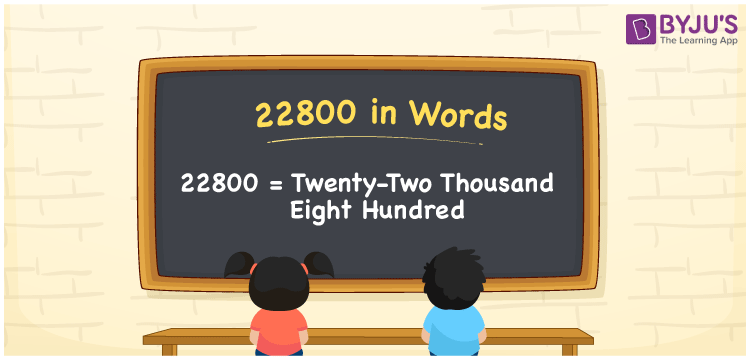# 22800 in Words

22800 in words can be written as Twenty-Two Thousand Eight Hundred. This article will help you learn how numbers can be written into words using the English alphabet. For example, if you spend Rs. 22800 to donate food at the orphanage, then you can say that “I spent Twenty-Two Thousand Eight Hundred Rupees to donate food at the orphanage”. The numbers in words concept is designed by the faculty after conducting a vast research on each concept. Hence, 22800 can be read as “Twenty-Two Thousand Eight Hundred” in English.

 22800 in words Twenty-Two Thousand Eight Hundred Twenty-Two Thousand Eight Hundred in Numbers 22800

## 22800 in English Words## How to Write 22800 in Words?

The place value chart of 22800 is well explained in this section for a good understanding of the concept among the students. In 22800, there are five digits and their place value can be learnt from the table below.

 Ten Thousands Thousands Hundreds Tens Ones 2 2 8 0 0

The expanded form of 22800 is:

2 x Ten Thousand + 2 x Thousand + 8 × Hundred + 0 × Ten + 0 × One

= 2 x 10000 + 2 x 1000 + 8 × 100 + 0 × 10 + 0 × 1

= 20000 + 2000 + 800

= 22800

= Twenty Two Thousand Eight Hundred

Therefore, 22800 in words is written as Twenty-Two Thousand Eight Hundred.

22800 is a natural number that precedes 22801 and succeeds 22799.

22800 in words – Twenty-two Thousand Eight Hundred

Is 22800 an odd number? – No

Is 22800 an even number? – Yes

Is 22800 a perfect square number? – No

Is 22800 a perfect cube number? – No

Is 22800 a prime number? – No

Is 22800 a composite number? – Yes

## Frequently Asked Questions on 22800 in Words

Q1

### How to write 22800 in words?

22800 can be written in words as “Twenty-Two Thousand Eight Hundred”.
Q2

### How can Twenty-Two Thousand Eight Hundred be written in numbers?

Twenty-Two Thousand Eight Hundred can be written in numbers as 22800.
Q3

### Is 22800 a perfect cube number?

No, 22800 is not a perfect cube number as it is not the product of three similar numbers.